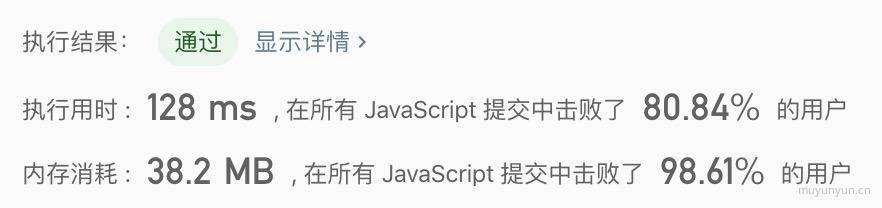Time FlyingYou are given two non-empty linked lists representing two non-negative integers. `The most significant digit comes first` and each of their nodes contain a single digit. Add the two numbers and return it as a linked list.

You may assume the two numbers do not contain any leading zero, except the number 0 itself.

What if you cannot modify the input lists? In other words, reversing the lists is not allowed.

Example:

``````Input: (7 -> 2 -> 4 -> 3) + (5 -> 6 -> 4)
Output: 7 -> 8 -> 0 -> 7``````

### Analyze

• 第一步: 首先补齐位数, 让其一一对应;
``````7  ->  2  ->  4  ->  3
0  ->  5  ->  6  ->  4``````
• 第二步: 递归计算两个链表同位之和, 同时使用 digitCarry 表示进位的情况;
``````/**
* function ListNode(val) {
*     this.val = val;
*     this.next = null;
* }
*/
/**
* @param {ListNode} l1
* @param {ListNode} l2
* @return {ListNode}
*/
var addTwoNumbers = function(l1, l2) {
let countl1 = 0, countl2 = 0
let l1List = l1
let l2List = l2
while(l1List) {
countl1++
l1List = l1List.next
}

while(l2List) {
countl2++
l2List = l2List.next
}

// creat the frontest List
let tmpList = new ListNode(0)
let cur = tmpList
let diff = Math.abs(countl2 - countl1)
while (diff--) {
cur.next = new ListNode(0)
cur = cur.next
}

if (countl1 < countl2) {
cur.next = l1
l1 = tmpList.next
} else if (countl2 < countl1) {
cur.next = l2
l2 = tmpList.next
}

// flag: 1 shows digit carry, 0 not;
let digitCarry = 0

/**
* calculate the sum of l1 and l2
*/
if (l1 === null) return

let sum = l1.val + l2.val + digitCarry
if (sum >= 10) {
l1.val = sum % 10
digitCarry = 1
} else {
l1.val = sum
digitCarry = 0
}
}

let result = l1
if (digitCarry === 1) {
result = new ListNode(1)
result.next = l1
}

return result
}``````2